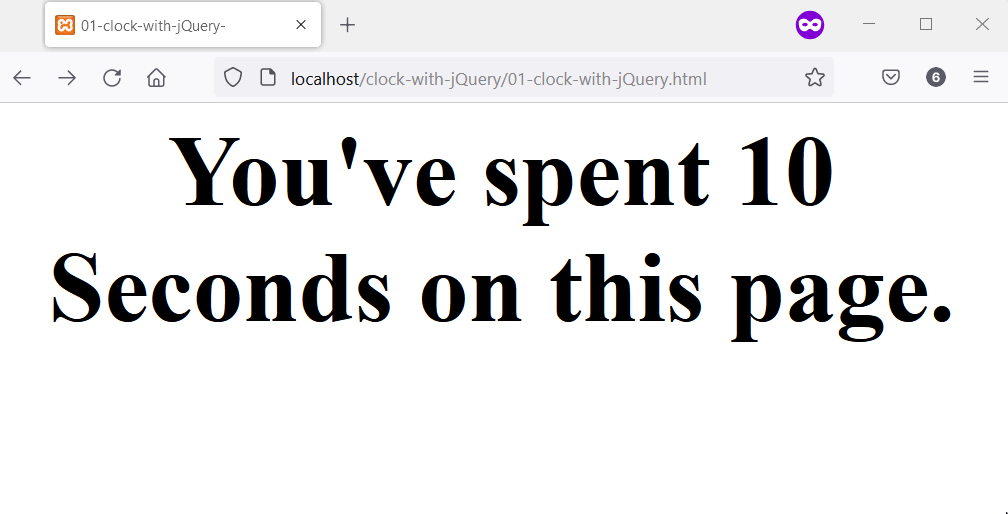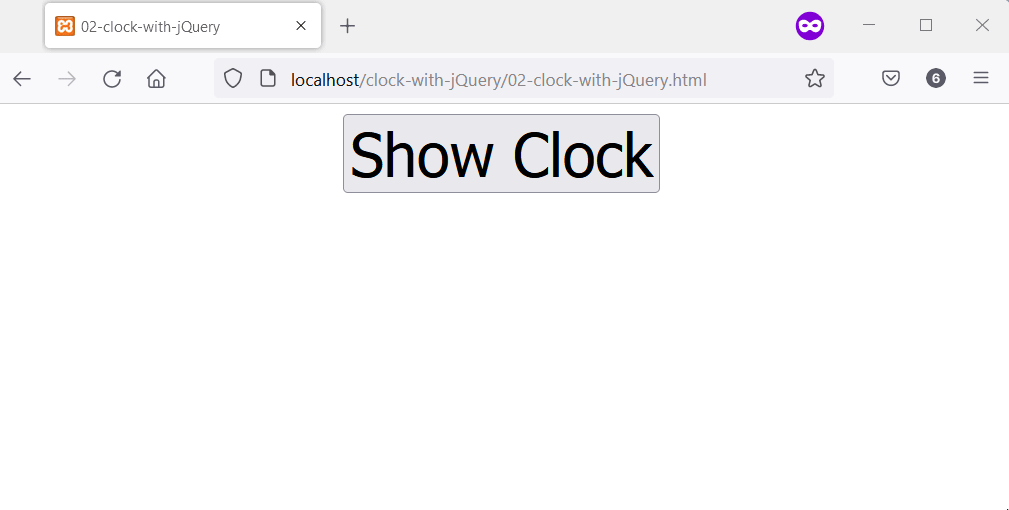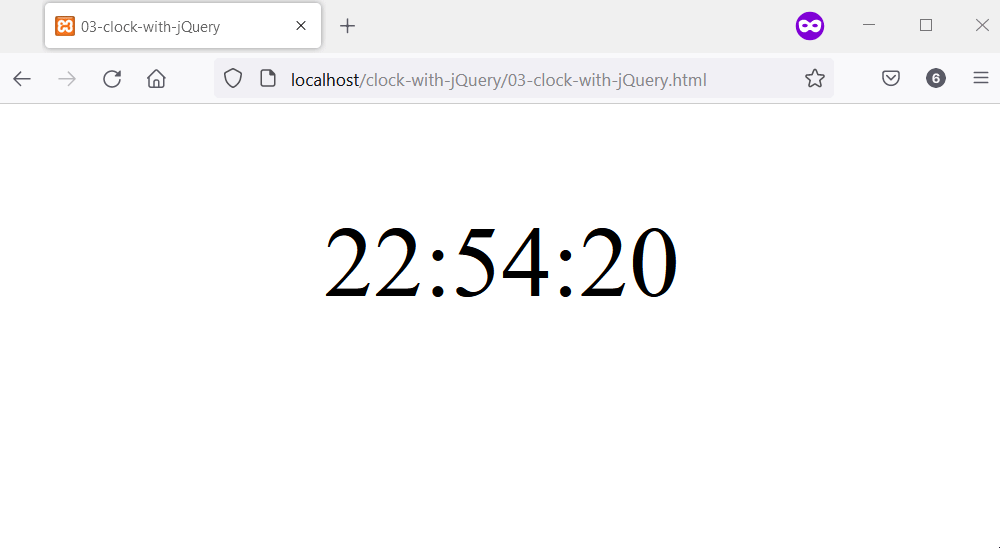# Create a Timer and a Clock Using jQuery

Habdul Hazeez Sep 19, 2022

This article introduces three examples of creating a timer and a clock in jQuery. The first example is a timer that starts once you open the page, while the second and third examples contain a clock implemented differently.

## Create a Timer in jQuery

To create a timer in jQuery you’ll need the `Date` object, `setInterval()`, `Math.round()`, and jQuery `text()` API. The `Date` object allows you to calculate the time difference in seconds.

At the same time, the `setInterval()` function will run the calculations at all times. As a result, the timer will show when you launch the script in your web browser.

The job of jQuery `text()` API is to place the timer in a selected HTML element. Finally, the `Math.round()` function allows the timer to show as integers, not decimal values.

``````<!DOCTYPE html>
<html lang="en">
<title>01-clock-with-jQuery-</title>
<body>
<div style="text-align: center; font-size: 5em;">
<b class="jquery_timer"></b>
</div>
<script>
// Create a new date object.
var date_object = new Date;
// Use setInterval to run a custom function
// that displays the timer at all times.
setInterval(function() {
// Additional information to aid user experience.
let prepend_text = "You've spent ";
// Use Math.round() to remove any decimal
// number from the timer.
\$('.jquery_timer').text(prepend_text + Math.round((new Date - date_object) / 1000) + " Seconds" + append_text);
}, 1000);
</script>
</body>
</html>
``````

Output:## Create a Clock in jQuery Using `setTimeout` and jQuery `text()` API

The core of this clock is a custom function that uses the `Date` object to calculate the date. To do this, you’ll use the `getHours()`, `getMinutes()`, and `getSeconds()` methods of the `Date` object.

When combined, these three methods will let you display the clock’s hours, minutes, and seconds. Afterward, you’ll use jQuery `text()` API to place the clock in an HTML element.

Before that, you’ll call `setTimeout()` on the custom function, which will run at regular intervals. The latter should be small enough and not cause the time to display inaccurate time.

Our code example below has implemented the clock using all the information. You’ll show the clock after clicking the HTML button to make it interesting.

``````<!DOCTYPE html>
<html lang="en">
<title>02-clock-with-jQuery</title>
<style>
button {
cursor: pointer;
}
</style>
<body>
<main style="text-align: center; font-size: 3em;">
<button class="show_clock" style="font-size: 1em;">Show Clock</button>
<h1 class="clock_location"></h1>
</main>
<script>
function get_current_date(){
let current_date = new Date();
let hours = current_date.getHours();
let minutes = current_date.getMinutes();
let seconds = current_date.getSeconds();
// they are less than 10
if (seconds < 10){
seconds = "0" + seconds;
}

if (minutes < 10) {
minutes = "0" + minutes;
}

\$(".clock_location").text(hours+" : "+minutes+" : "+seconds);
setTimeout(function(){
get_current_date()
}, 500);
}
// Wait for the user to click the button
// before you show the clock.
\$(".show_clock").click(get_current_date);
});
</script>
</body>
</html>
``````

Output:## Create a Clock in jQuery Using `setInterval` and jQuery `html()` API

Like our previous example, you’ll create a clock using jQuery, but differently. That’s because, here, you’ll use the `setInterval()` function and jQuery `html()` API.

At first, you’ll call the `setInterval()` function on an anonymous function. This function will calculate and show the date in your web browser.

The function will use the `Date` object to get the current hour, minutes, and seconds. You can add leading zeros to the clock parts and compose them for display.

Afterward, use jQuery to grab an HTML element where you’ll like to display the clock. The final step is to call jQuery `html()` API on the composed time and show it within the HTML element.

``````<!DOCTYPE html>
<html lang="en">
<title>03-clock-with-jQuery</title>
<body>
<main style="text-align: center; font-size: 5em;">
<p class="clock_location"></p>
</main>
<script>
// Use setInterval to run an anonymous function
// every one milliseconds.
setInterval(function() {
let current_date = new Date();
let clock_hours = current_date.getHours();
let clock_minutes = current_date.getMinutes();
let clock_seconds = current_date.getSeconds();
// zeros to elements of the clock if they
// are less than 10.
if (clock_hours < 10) {
clock_hours = "0" + clock_hours;
}

if (clock_minutes < 10) {
clock_minutes = "0" + clock_minutes;
}

if (clock_seconds < 10){
clock_seconds = "0" + clock_seconds;
}
// Compose the current time
let clock_delimeter = ":";
let current_time = clock_hours + clock_delimeter + clock_minutes + clock_delimeter + clock_seconds;
\$(".clock_location").html(current_time);
}, 1000);
</script>
</body>
</html>
``````

Output:Habdul Hazeez is a technical writer with amazing research skills. He can connect the dots, and make sense of data that are scattered across different media.